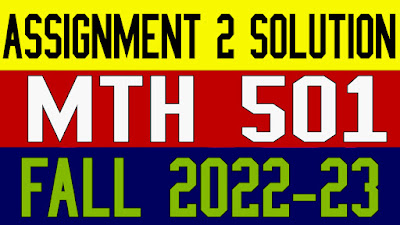### Latest Post

6/recent/ticker-posts

# Mth501 Assignment 2 Solution Fall 2022

MTH501 Assignment 2 Solution Fall 2022: Today we are sharing with you mth501 assignment 2 solution fall 2022 before creating your assignment & gdb file please read all the instructions carefully and follow the rules.Mth501 Assignment 2 Solution Fall 2022

Algebra is a branch of Mathematics that uses mathematical statements to describe relationships between things that vary over time. These variables include things like the relationship between supply of an object and its price. When we use a mathematical statement to describe a relationship, we often use letters to represent the quantity that varies, since it is not a fixed amount. These letters and symbols are referred to as variables.

In algebra, letters are sometimes used in place of numbers. The mathematical statements that describe relationships are expressed using algebraic terms, expressions, or equations (mathematical statements containing letters or symbols to represent numbers). Before we use algebra to find information about these kinds of relationships, it is important to first introduce some basic terminology.

Mth501 Assignment 2 Solution Fall 2022

Question No 1:

Find the determinant of the given matrix A by using both row reduction and cofactor expansion.
Check Whether the matrix A is strictly diagonally dominant or not.